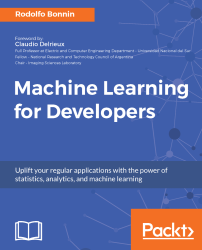•#### Machine Learning for Developers#### Overview of this book

Most of us have heard about the term Machine Learning, but surprisingly the question frequently asked by developers across the globe is, “How do I get started in Machine Learning?”. One reason could be attributed to the vastness of the subject area because people often get overwhelmed by the abstractness of ML and terms such as regression, supervised learning, probability density function, and so on. This book is a systematic guide teaching you how to implement various Machine Learning techniques and their day-to-day application and development. You will start with the very basics of data and mathematical models in easy-to-follow language that you are familiar with; you will feel at home while implementing the examples. The book will introduce you to various libraries and frameworks used in the world of Machine Learning, and then, without wasting any time, you will get to the point and implement Regression, Clustering, classification, Neural networks, and more with fun examples. As you get to grips with the techniques, you’ll learn to implement those concepts to solve real-world scenarios for ML applications such as image analysis, Natural Language processing, and anomaly detections of time series data. By the end of the book, you will have learned various ML techniques to develop more efficient and intelligent applications.
PrefaceFree Chapter
Introduction - Machine Learning and Statistical ScienceThe Learning ProcessClusteringLinear and Logistic RegressionNeural NetworksConvolutional Neural NetworksRecurrent Neural NetworksRecent Models and DevelopmentsSoftware Installation and Configuration# Univariate time series prediction with energy consumption data

In this example, we will be solving a problem in the domain of regression. For this reason, we will build a multi-layer RNN with two LSTMs. The type of regression we will do is of the many to one type, because the network will receive a sequence of energy consumption values and will try to output the next value based on the previous four registers.

The dataset we will be working on is a compendium of many measurements of the power consumption of one home over a period of time. As we might infer, this kind of behavior can easily follow patterns (it increases when the occupants use the microwave to prepare breakfast and use computers during the day, it decreases a bit in the afternoon, and then increases in the evening with all the lights, finally decreasing to zero when the occupants are asleep).

Let's start by...